# Two chords

In a circle with radius r = 26 cm two parallel chords are drawn. One chord has a length t1 = 48 cm and the second has a length t2 = 20 cm, with the center lying between them. Calculate the distance of two chords.

v1 =  34 cm
v2 =  14 cm

### Step-by-step explanation: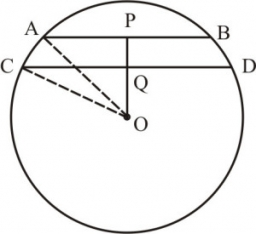Did you find an error or inaccuracy? Feel free to write us. Thank you!Tips to related online calculators
Do you want to convert length units?
Pythagorean theorem is the base for the right triangle calculator.

#### You need to know the following knowledge to solve this word math problem:

We encourage you to watch this tutorial video on this math problem:

## Related math problems and questions:

• Two parallel chordsIn a circle 70 cm in diameter, two parallel chords are drawn so that the center of the circle lies between the chords. Calculate the distance of these chords if one of them is 42 cm long and the second 56 cm.
• Two chords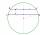In a circle with a radius of 8.5 cm, two parallel chords are constructed, the lengths of which are 9 cm and 12 cm. Find the distance of the chords in a circle.
• Two parallel chords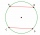The two parallel chords of the circle have the same length of 6 cm and are 8 cm apart. Calculate the radius of the circle.
• CirclesIn the circle with a radius 7.5 cm are constructed two parallel chord whose lengths are 9 cm and 12 cm. Calculate the distance of these chords (if there are two possible solutions write both).
• Length of the chord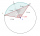Calculate the length of the chord in a circle with a radius of 25 cm with a central angle of 26°.
• Chors centers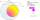The circle with a diameter 17 cm, upper chord /CD/ = 10.2 cm and bottom chord /EF/ = 7.5 cm. The midpoints of the chords H, G is that /EH/ = 1/2 /EF/ and /CG/ = 1/2 /CD/. Determine the distance between the G and H, if CD II EF (parallel).
• Common chordTwo circles with radius 17 cm and 20 cm are intersect at two points. Its common chord is long 27 cm. What is the distance of the centers of these circles?
• ChordIn a circle with radius r=60 cm is chord 4× longer than its distance from the center. What is the length of the chord?
• Chord distanceThe circle k (S, 6 cm), calculate the chord distance from the center circle S when the length of the chord is t = 10 cm.
• Circle's chordsIn the circle there are two chord length 30 and 34 cm. The shorter one is from the center twice than longer chord. Determine the radius of the circle.
• Two chordsCalculate the length of chord AB and perpendicular chord BC to circle if AB is 4 cm from the center of the circle and BC 8 cm from the center of the circle.
• Circle chordCalculate the length of the chord of the circle with radius r = 10 cm, length of which is equal to the distance from the center of the circle.
• Chord 2Point A has a distance of 13 cm from the center of the circle with a radius r = 5 cm. Calculate the length of the chord connecting the points T1 and T2 of contact of tangents led from point A to the circle.
• Chord MNChord MN of circle has distance from the center circle S 120 cm. Angle MSN is 64°. Determine the radius of the circle.
• Chord 4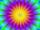I need to calculate the circumference of a circle, I know the chord length c=22 cm and the distance from the center d=29 cm chord to the circle.
• The chordCalculate a chord length which the distance from the center of the circle (S, 6 cm) equals 3 cm.
• Chord ABWhat is the chord AB's length if its distance from the center S of the circle k(S, 92 cm) is 10 cm?